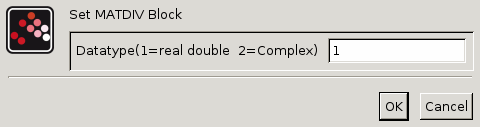Scilab Home page | Wiki | Bug tracker | Forge | Mailing list archives | ATOMS | File exchange
Change language to: English - Français - Português - Русский
Scilabヘルプ >> Xcos > palettes > Matrix operation palette > MATDIV

# MATDIV

Matrix division

### Block Screenshot### Description

The MATDIV block outputs the right matrix division. It is a solution to x*B=A. The higher input is the A matrix, the lower one is the B matrix, and the output is x.

If A is an M1xN matrix, B must be a M2xN where M1 and M2 can be different or equal. The output x is a M1xM2 matrix.

The equivalent of MATDIV is slash (/) in Scilab.

### Parameters• Datatype(1=real double 2=Complex)

It indicates the type of the output. It support only the two types double (1) and complex (2). If we input another entry in this label Xcos will print the message "Datatype is not supported".

Properties : Type 'vec' of size 1.

### Default properties

• always active: no

• direct-feedthrough: yes

• zero-crossing: no

• mode: no

• regular inputs:

- port 1 : size [-1,-3] / type 1

- port 2 : size [-2,-3] / type 1

• regular outputs:

- port 1 : size [-1,-2] / type 1

• number/sizes of activation inputs: 0

• number/sizes of activation outputs: 0

• continuous-time state: no

• discrete-time state: no

• object discrete-time state: no

• name of computational function: mat_div

### Example

```A = [1 7 3 ; 23 32 29]
B = [21 18 34 ; 13 10 19 ; 11 54 36]
// then the result of the x*B = A equation is (A/B):
x = [-0.475 0.712 0.156 ; -4.350 8.381 0.491]```

Division example: [t sin(t)]/[0 1; 1 0] = [sin(t) t]### Interfacing function

• SCI/modules/scicos_blocks/macros/MatrixOp/MATDIV.sci

### Computational function

• SCI/modules/scicos_blocks/src/c/mat_div.c
• SCI/modules/scicos_blocks/src/c/matz_div.c